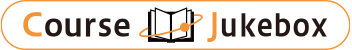### CourseDetailDegree
Bachelor
1
Course delivery methods
Subject
Physical sciences, Mathematical sciences, Engineering & technology
Program
School
School of Science and Engineering
Department
Bachelor's Program in Interdisciplinary Engineering
Campus
Tsukuba Campus
Classroom
3A213
Course Offering Year
2023-2024
Course Offering Month
April - August
Weekday and Period
Wed4,5
Capacity
Credits
3.0
Language
English
Course Number
FJ20014

### Linear Algebra II University of Tsukuba

#### Course Overview

Following “Linear Algebra I”’, “Linear Algebra II” will also concentrate on the basics of linear algebra. Emphasis will be given to topics that
will be useful in other disciplines, such as determinants, eigenvalues,
positive definite matrices, Fourier series and the Fast Fourier
Transform. Some homework problems may require you to use a program such as MATLAB or Mathematica, an important tool for numerical linear algebra.
No previous programming experience is required.

#### Learning Achievement

Following “Linear Algebra I”’, “Linear Algebra II” will also concentrate on the basics of linear algebra. Emphasis will be given to topics that will be useful in other disciplines, such as determinants, eigenvalues, positive definite matrices, Fourier series and the Fast Fourier Transform. Some homework problems may require you to use a program such as MATLAB or Mathematica, an important tool for numerical linear algebra. No previous programming experience is required.

#### Competence

Related to 1. Mathematical logic and calculation skills.

#### Course prerequisites

“Linear Algebra I” course

Homework 40 %
Midterm Exam 30 %
Final Exam 30 %

#### Course schedule

Properties of determinants
Properties of determinants
Eigenvalues and eigenvectors
Eigenvalues and eigenvectors
Symmetric matrices and positive definite matrices
Symmetric matrices and positive definite matrices
Singular Value Decomposition (SVD)
Singular Value Decomposition (SVD)
Linear transformations and change of basis
Linear transformations and change of basis
Similar matrices and Jordan form
Similar matrices and Jordan form
Complex matrices and Fast Fourier transform
Complex matrices and Fast Fourier transform
Markov matrices and Fourier series
Markov matrices and Fourier series
Linear programming
Linear programming

#### Course type

Lectures and Class Exercises

Sharmin Sonia

#### Other information

The homework is essential in learning linear algebra. You are encouraged to talk to other students about difficult problems. But you must write your own solutions.
There will be a midterm one-hour exam at class times and a final exam. The use of calculators or notes is not permitted during the exams.

#### Site for Inquiry

Link to the syllabus provided by the university